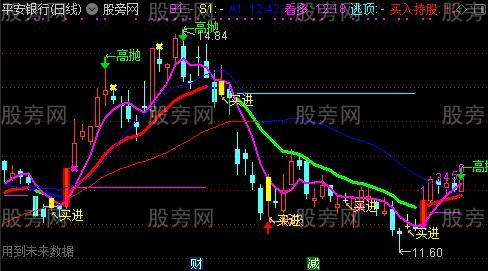# 通达信九转变异主图指标公式

Ema13:=EMA(C,13),COLORWHITE;
EMA21:=EMA(C,21),COLORYELLOW;
EMA34:=EMA(C,34),COLORFF00FF;
EMA60:=MA(C,60),COLORFFCC66;
VAR2:=(2*CLOSE+HIGH+LOW)/4;
VAR3:=IF(YEAR>=2099 AND MONTH>2,0,1);
VAR4:=LLV(LOW,5); VAR5:=HHV(HIGH,4);

DRAWTEXT(crOSS(散户,庄家) AND 散户<30,L-0.05,'←低吸'),COLORLIRED;
DRAWTEXT(CROSS(庄家,散户) AND 散户>70,H+0.05,'←高抛'),COLORGREEN;
DRAWICON(CROSS(散户,庄家) AND 散户<30,L-0.05,1);
DRAWICON(CROSS(庄家,散户) AND 散户>70,H+0.05,2);
VAR9:=CLOSE-REF(CLOSE,5);
VARA:=EMA(EMA(VAR9,5),5)/EMA(EMA(Abs(VAR9),5),5);
VARC:=EMA(EMA(VAR9,20),20)/EMA(EMA(ABS(VAR9),20),20);

DRAWICON(卖出<0,H+0.05,2); 买线:=EMA(CLOSE,2);

B1:IF(CROSS(买线,卖线),L-0.02,DRAWNULL),CROSSDOT,LINETHICK3,COLORMAGENTA;
S1:IF(CROSS(80,rsi)*30,H+0.02,DRAWNULL),CROSSDOT,LINETHICK3,COLORYELLOW;
VC:=((CLOSE-MA(CLOSE,6))/MA(CLOSE,6)*100+(CLOSE-MA(CLOSE,24))/MA(CLOSE,24)*100+(CLOSE-MA (CLOSE,32))/MA(CLOSE,32)*100)/3;
S2:=IF(VC>20 AND FILTER(VC< REF(VC,1),5),H+0.02,DRAWNULL),CIRCLEDOT,LINETHICK3,COLORGREEN;
A0:=(L+H+C*2)/4;
A1:EMA(A0,14)COLORBLUE,LINETHICK2;
A2:=EMA(A0,25)COLORLIBLUE,LINETHICK2;
A1X:=(A1-REF(A1,1))/REF(A1,1)*100,NODRAW;
A2X:=(A2-REF(A2,1))/REF(A2,1)*100,NODRAW;

STICKLINE(CROSS(A1X,0),H,L,0,0 ),COLOR9933FF;
STICKLINE(CROSS(A1X,0),C,O,3,0 ),COLOR0000CC;
STICKLINE(CROSS(A1X,0),C,O,1,0 ),COLOR0033FF;
STICKLINE(CROSS(0,A1X),L,H,0,0 ),COLOR66CC33;
N:=CURRBARSCOUNT;
{买入结构}
B:=C<REF(C,4);
DRAWNUMBER(N=6 AND REFXV(COUNT(B,6),5)=6 AND REF(B,1)=0,L,1),COLORMAGENTA;
DRAWNUMBER(N=7 AND REFXV(COUNT(B,7),6)=7 AND REF(B,1)=0,L,1),COLORMAGENTA;
DRAWNUMBER(N=8 AND REFXV(COUNT(B,8),7)=8 AND REF(B,1)=0,L,1),COLORMAGENTA;
DRAWNUMBER(N>=9 AND REFXV(COUNT(B,9),8)=9 AND REF(B,1)=0,L,1),COLORMAGENTA;
DRAWNUMBER(N=5 AND REFXV(COUNT(B,5),4)=5 AND COUNT(B,2)=2 AND REF(B,1)=0,L,2),COLORMAGENTA;
DRAWNUMBER(N=6 AND REFXV(COUNT(B,6),5)=6 AND COUNT(B,2)=2 AND REF(B,1)=0,L,2),COLORMAGENTA;
DRAWNUMBER(N=7 AND REFXV(COUNT(B,7),6)=7 AND COUNT(B,2)=2 AND REF(B,1)=0,L,2),COLORMAGENTA;
DRAWNUMBER(N>=8 AND REFXV(COUNT(B,8),7)=8 AND COUNT(B,2)=2 AND REF(B,2)=0,L,2),COLORMAGENTA;
DRAWNUMBER(N=4 AND REFXV(COUNT(B,4),3)=4 AND COUNT(B,3)=3 AND REF(B,3)=0,L,3),COLORMAGENTA;
DRAWNUMBER(N=5 AND REFXV(COUNT(B,5),4)=5 AND COUNT(B,3)=3 AND REF(B,3)=0,L,3),COLORMAGENTA;
DRAWNUMBER(N=6 AND REFXV(COUNT(B,6),5)=6 AND COUNT(B,3)=3 AND REF(B,3)=0,L,3),COLORMAGENTA;
DRAWNUMBER(N>=7 AND REFXV(COUNT(B,7),6)=7 AND COUNT(B,3)=3 AND REF(B,3)=0,L,3),COLORMAGENTA;
DRAWNUMBER(N=3 AND REFXV(COUNT(B,3),2)=3 AND COUNT(B,4)=4 AND REF(B,4)=0,L,4),COLORMAGENTA;
DRAWNUMBER(N=4 AND REFXV(COUNT(B,4),3)=4 AND COUNT(B,4)=4 AND REF(B,4)=0,L,4),COLORMAGENTA;
DRAWNUMBER(N=5 AND REFXV(COUNT(B,5),4)=5 AND COUNT(B,4)=4 AND REF(B,4)=0,L,4),COLORMAGENTA;
DRAWNUMBER(N>=6 AND REFXV(COUNT(B,6),5)=6 AND COUNT(B,4)=4 AND REF(B,4)=0,L,4),COLORMAGENTA;
DRAWNUMBER(N=2 AND REFXV(COUNT(B,2),1)=2 AND COUNT(B,5)=5 AND REF(B,5)=0,L,5),COLORMAGENTA;
DRAWNUMBER(N=3 AND REFXV(COUNT(B,3),2)=3 AND COUNT(B,5)=5 AND REF(B,5)=0,L,5),COLORMAGENTA;
DRAWNUMBER(N=4 AND REFXV(COUNT(B,4),3)=4 AND COUNT(B,5)=5 AND REF(B,5)=0,L,5),COLORMAGENTA;
DRAWNUMBER(N>=5 AND REFXV(COUNT(B,5),4)=5 AND COUNT(B,5)=5 AND REF(B,5)=0,L,5),COLORMAGENTA;
DRAWNUMBER(N=1 AND COUNT(B,6)=6 AND REF(B,6)=0,L,6),COLORMAGENTA;
DRAWNUMBER(N=2 AND REFXV(COUNT(B,2),1)=2 AND COUNT(B,6)=6 AND REF(B,6)=0,L,6),COLORMAGENTA;
DRAWNUMBER(N=3 AND REFXV(COUNT(B,3),2)=3 AND COUNT(B,6)=6 AND REF(B,6)=0,L,6),COLORMAGENTA;
DRAWNUMBER(N>=4 AND REFXV(COUNT(B,4),3)=4 AND COUNT(B,6)=6 AND REF(B,6)=0,L,6),COLORMAGENTA;
DRAWNUMBER(N=1 AND COUNT(B,7)=7 AND REF(B,7)=0,L,7),COLORMAGENTA;
DRAWNUMBER(N=2 AND REFXV(COUNT(B,3),1)=3 AND COUNT(B,7)=7 AND REF(B,7)=0,L,7),COLORMAGENTA;
DRAWNUMBER(N>=3 AND REFXV(COUNT(B,3),2)=3 AND COUNT(B,7)=7 AND REF(B,7)=0,L,7),COLORMAGENTA;
DRAWNUMBER(N=2 AND COUNT(B,8)=8 AND REF(B,8)=0,L,8),COLORMAGENTA;
DRAWNUMBER(N>=2 AND REFXV(COUNT(B,2),1)=2 AND COUNT(B,8)=8 AND REF(B,8)=0,L,8),COLORMAGENTA;
DRAWNUMBER(N>=1 AND COUNT(B,9)=9 AND REF(B,9)=0,L,9),COLORGREEN;
{卖出结构}
S:=C>REF(C,4);
DRAWNUMBER(N=6 AND REFXV(COUNT(S,6),5)=6 AND REF(S,1)=0,H*1.01,1),COLORMAGENTA;
DRAWNUMBER(N=7 AND REFXV(COUNT(S,7),6)=7 AND REF(S,1)=0,H*1.01,1),COLORMAGENTA;
DRAWNUMBER(N=8 AND REFXV(COUNT(S,8),7)=8 AND REF(S,1)=0,H*1.01,1),COLORMAGENTA;
DRAWNUMBER(N>=9 AND REFXV(COUNT(S,9),8)=9 AND REF(S,1)=0,H*1.01,1),COLORMAGENTA;
DRAWNUMBER(N=5 AND REFXV(COUNT(S,5),4)=5 AND COUNT(S,2)=2 AND REF(S,1)=0,H*1.01,2),COLORMAGENTA;
DRAWNUMBER(N=6 AND REFXV(COUNT(S,6),5)=6 AND COUNT(S,2)=2 AND REF(S,1)=0,H*1.01,2),COLORMAGENTA;
DRAWNUMBER(N=7 AND REFXV(COUNT(S,7),6)=7 AND COUNT(S,2)=2 AND REF(S,1)=0,H*1.01,2),COLORMAGENTA;
DRAWNUMBER(N>=8 AND REFXV(COUNT(S,8),7)=8 AND COUNT(S,2)=2 AND REF(S,2)=0,H*1.01,2),COLORMAGENTA;
DRAWNUMBER(N=4 AND REFXV(COUNT(S,4),3)=4 AND COUNT(S,3)=3 AND REF(S,3)=0,H*1.01,3),COLORMAGENTA;
DRAWNUMBER(N=5 AND REFXV(COUNT(S,5),4)=5 AND COUNT(S,3)=3 AND REF(S,3)=0,H*1.01,3),COLORMAGENTA;
DRAWNUMBER(N=6 AND REFXV(COUNT(S,6),5)=6 AND COUNT(S,3)=3 AND REF(S,3)=0,H*1.01,3),COLORMAGENTA;
DRAWNUMBER(N>=7 AND REFXV(COUNT(S,7),6)=7 AND COUNT(S,3)=3 AND REF(S,3)=0,H*1.01,3),COLORMAGENTA;
DRAWNUMBER(N=3 AND REFXV(COUNT(S,3),2)=3 AND COUNT(S,4)=4 AND REF(S,4)=0,H*1.01,4),COLORMAGENTA;
DRAWNUMBER(N=4 AND REFXV(COUNT(S,4),3)=4 AND COUNT(S,4)=4 AND REF(S,4)=0,H*1.01,4),COLORMAGENTA;
DRAWNUMBER(N=5 AND REFXV(COUNT(S,5),4)=5 AND COUNT(S,4)=4 AND REF(S,4)=0,H*1.01,4),COLORMAGENTA;
DRAWNUMBER(N>=6 AND REFXV(COUNT(S,6),5)=6 AND COUNT(S,4)=4 AND REF(S,4)=0,H*1.01,4),COLORMAGENTA;
DRAWNUMBER(N=2 AND REFXV(COUNT(S,2),1)=2 AND COUNT(S,5)=5 AND REF(S,5)=0,H*1.01,5),COLORMAGENTA;
DRAWNUMBER(N=3 AND REFXV(COUNT(S,3),2)=3 AND COUNT(S,5)=5 AND REF(S,5)=0,H*1.01,5),COLORMAGENTA;
DRAWNUMBER(N=4 AND REFXV(COUNT(S,4),3)=4 AND COUNT(S,5)=5 AND REF(S,5)=0,H*1.01,5),COLORMAGENTA;
DRAWNUMBER(N>=5 AND REFXV(COUNT(S,5),4)=5 AND COUNT(S,5)=5 AND REF(S,5)=0,H*1.01,5),COLORMAGENTA;
DRAWNUMBER(N=1 AND COUNT(S,6)=6 AND REF(S,6)=0,H*1.01,6),COLORMAGENTA;
DRAWNUMBER(N=2 AND REFXV(COUNT(S,2),1)=2 AND COUNT(S,6)=6 AND REF(S,6)=0,H*1.01,6),COLORMAGENTA;
DRAWNUMBER(N=3 AND REFXV(COUNT(S,3),2)=3 AND COUNT(S,6)=6 AND REF(S,6)=0,H*1.01,6),COLORMAGENTA;
DRAWNUMBER(N>=4 AND REFXV(COUNT(S,4),3)=4 AND COUNT(S,6)=6 AND REF(S,6)=0,H*1.01,6),COLORMAGENTA;
DRAWNUMBER(N=1 AND COUNT(S,7)=7 AND REF(S,7)=0,H*1.01,7),COLORMAGENTA;
DRAWNUMBER(N=2 AND REFXV(COUNT(S,3),1)=3 AND COUNT(S,7)=7 AND REF(S,7)=0,H*1.01,7),COLORMAGENTA;
DRAWNUMBER(N>=3 AND REFXV(COUNT(S,3),2)=3 AND COUNT(S,7)=7 AND REF(S,7)=0,H*1.01,7),COLORMAGENTA;
DRAWNUMBER(N=2 AND COUNT(S,8)=8 AND REF(S,8)=0,H*1.01,8),COLORMAGENTA;
DRAWNUMBER(N>=2 AND REFXV(COUNT(S,2),1)=2 AND COUNT(S,8)=8 AND REF(S,8)=0,H*1.01,8),COLORMAGENTA;
DRAWNUMBER(N>=1 AND COUNT(S,9)=9 AND REF(S,9)=0,H*1.01,9),COLORYELLOW;
MAA5:MA(C,5),COLORFF00FF,LINETHICK2;
MAA10:=MA(C,10);
MAA30:MA(C,30),COLORRED;
KS:IF(MAA10>MAA30,MAA10,MAA30),COLORBLUE;
A3:=(C-LLV(L,9))/(HHV(H,9)-LLV(L,9))*100;
A4:=SMA(A3,3,1);
A5:=SMA(A4,3,1);
A6:=3*A4-2*A5;
A7:=C-REF(C,1);
A8:=100*EMA(EMA(A7,6),6)/EMA(EMA(ABS(A7),6),6);

DRAWTEXT(FILTER(买=1,5),LOW-0.05,'↖买进'),COLORYELLOW;
STICKLINE(买,OPEN,CLOSE,2,0),COLORYELLOW;### 相关文章